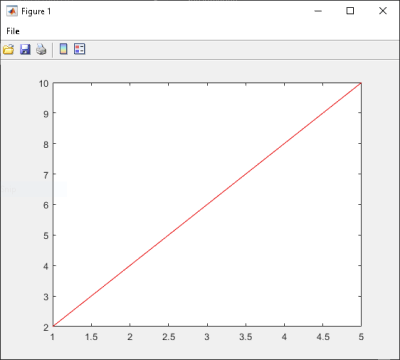Integrate Function with Variable Number of Arguments

This example shows you how to create a .NET application using a MATLAB® function that takes a variable number of arguments instead of just one.

In this example, you perform the following steps:

• Use the MATLAB Compiler SDK™ product to convert the MATLAB function drawgraph to a method of a .NET class (Plotter) and wrap the class in a .NET assembly (VarArgComp). The drawgraph function displays a plot of the input parameters and is called as a method of the Plotter class.

• Access the component in a C# application (VarArgApp.cs) or a Visual Basic® application (VarArgApp.vb) by instantiating the Plotter class and using MWArray to represent data.

• Build and run the VarArgDemoApp application using the Visual Studio® .NET development environment.

Files

 MATLAB Functions drawgraph.m extractcoords.m MATLAB Function Location matlabroot\toolbox\dotnetbuilder\Examples\VSVersion\NET\VarArgExample\VarArgComp\ C# Code Location matlabroot\toolbox\dotnetbuilder\Examples\VSVersion\NET\VarArgExample\VarArgCSApp\VarArgApp.cs Visual Basic Code Location matlabroot\toolbox\dotnetbuilder\Examples\VSVersion\NET\VarArgExample\VarArgVBApp\VarArgApp.vb

Procedure

1. Copy the following folder that ships with the MATLAB product to your work folder:

matlabroot\toolbox\dotnetbuilder\Examples\VSVersion\NET\VarArgExample

At the MATLAB command prompt, navigate to the new VarArgExample\VarArgComp subfolder in your work folder.

2. Examine the drawgraph and extractcoords functions.

function [xyCoords] = DrawGraph(colorSpec, varargin)

numVarArgIn= length(varargin);
xyCoords= zeros(numVarArgIn, 2);

for idx = 1:numVarArgIn
xCoord = varargin{idx}(1);
yCoord = varargin{idx}(2);

x(idx) = xCoord;
y(idx) = yCoord;

xyCoords(idx,1) = xCoord;
xyCoords(idx,2) = yCoord;
end

xmin = min(0, min(x));
ymin = min(0, min(y));

axis([xmin fix(max(x))+3 ymin fix(max(y))+3])

plot(x, y, 'color', colorSpec);

function [varargout] = ExtractCoords(coords)

for idx = 1:nargout
varargout{idx}= coords(idx,:);
end

3. Build the .NET component with the Library Compiler app or compiler.build.dotNETAssembly using the following information:

FieldValue
Library NameVarArgComp
Class NamePlotter
Files to Compileextractcoords.m   drawgraph.m

For example, if you are using compiler.build.dotNETAssembly, type:

buildResults = compiler.build.dotNETAssembly(["extractcoords.m","drawgraph.m"], ...
'AssemblyName','VarArgComp', ...
'ClassName','Plotter');

For more details, see the instructions in Generate .NET Assembly and Build .NET Application.

4. Decide whether you are using C# or Visual Basic to access the component.

• C#

If you are using C#, write source code for a C# application that accesses the component.

The sample application for this example is in VarArgExample\VarArgCSApp\VarArgApp.cs.

The following statements are alternative ways to call the drawgraph method:

data= (MWNumericArray)plotter.drawgraph(colorSpec,
coords, coords, coords,coords, coords);
...
data= (MWNumericArray)plotter.drawgraph((MWArray)colorSpec, coords);

• Visual Basic

If you are using Visual Basic, write source code for a Visual Basic application that accesses the component.

The sample application for this example is in VarArgExample\VarArgVBApp\VarArgApp.vb.

The following statements are alternative ways to call the drawgraph method:

data = CType(plotter.drawgraph(colorSpec, coords(0), coords(1), coords(2),
coords(3), coords(4)), MWNumericArray)
...
data = CType(plotter.drawgraph(colorSpec, coords), MWNumericArray)

In either case, the VarArgApp program does the following:

• Initializes three arrays (colorSpec, data, and coords) using the MWArray class library

• Creates a Plotter object

• Calls the extracoords and drawgraph methods

• Uses MWNumericArray to represent the data needed by the methods

• Uses a try-catch block to catch and handle any exceptions

5. Open the .NET project file that corresponds to your application language using Visual Studio.

• C#

If you are using C#, the VarArgCSApp folder contains a Visual Studio .NET project file for this example. Open the project in Visual Studio .NET by double-clicking VarArgCSApp.csproj in Windows® Explorer. You can also open it from the desktop by right-clicking VarArgCSApp.csproj and selecting Open Outside MATLAB.

• Visual Basic

If you are using Visual Basic, the VarArgVBApp folder contains a Visual Studio .NET project file for this example. Open the project in Visual Studio .NET by double-clicking VarArgVBApp.vbproj in Windows Explorer. You can also open it from the desktop by right-clicking VarArgVBApp.vbproj and selecting Open Outside MATLAB.

6. Create a reference to your assembly file VarArgComp.dll located in the folder where you generated or installed the assembly.

7. Create a reference to the MWArray API.

 If MATLAB is installed on your system matlabroot\toolbox\dotnetbuilder\bin\win64\\MWArray.dll If MATLAB Runtime is installed on your system \toolbox\dotnetbuilder\bin\win64\\MWArray.dll

8. Build and run the VarArgApp application in Visual Studio .NET.

The program displays the following output:

result=
1     2
2     4
3     6
4     8
5    10